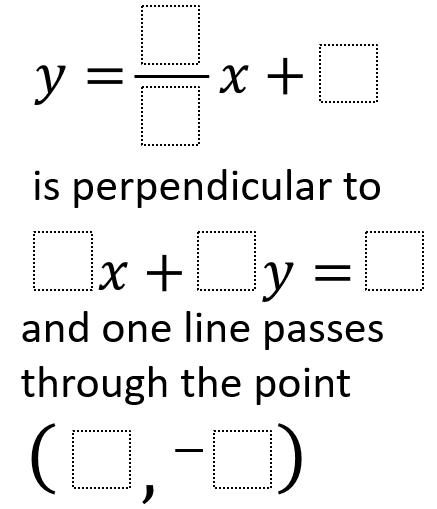Home > High School: Geometry > Expressing Geometric Properties with Equations > Perpendicular lines through a given point

# Perpendicular lines through a given point

Directions: Using the numbers 0 through 9, at most one time each, fill in the boxes to create a correct statment:### Hint

What do we need to have true for the lines to be perpendicular?
What would it mean for the equation of a line to pass through a given coordinate?

y=2/8x+6
4x+1y=5
(3,-7)

Another possible solution is:
6x+3y=9
y=(1/2)x+5
(4,-5)

Source: Andy Schwen

## Pythagorean Inequality

Directions: Use the whole numbers 1 through 6, at most one time each, to find …